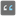• # Hint for solving number length

## Question related to missionNumber Length

Hello,

my solution for the "number length" task is

... return assert.length; ...

But for the number zero it shows me a 2 and I don't know why.

Can you give me a hint what I can do now? I'm a beginner.

My complete code:

import assert from "assert";

function numberLength(value: number): number {

```return assert.length;
```

}

console.log('Example:'); console.log(numberLength(10));

// These "asserts" are used for self-checking assert.equal(numberLength(10), 2); assert.equal(numberLength(0), 1); assert.equal(numberLength(4), 1); assert.equal(numberLength(44), 2);

console.log("Coding complete? Click 'Check' to earn cool rewards!");2